# barplot3d

## Introduction

One must be careful when using 3D plots of any kind. It is trivial to make them:

1. Cool

I hope this package and vignette enables the former and deters the latter.

## Package structure

There are a few functions in this package:

1. bar3d()
This adds a single 3D bar to the current RGL scene and is the basis of the whole package. You are unlikely to ever call this function.
2. barplot3d()
This is the workhorse function and calls bar3d() repeatedly to build your plot
3. legoplot() This is a wrapper around barplot3d() designed to draw a “legoplot”, which is used in the bioinformatics of DNA sequencing analysis

## Two example plots

### Something very simple

## Load packages
library(rgl)
library(barplot3d)
## Make a very simple 3D barplot using mostly defaults
barplot3d(rows=1,cols=5,z=1:5,theta=10,phi=10)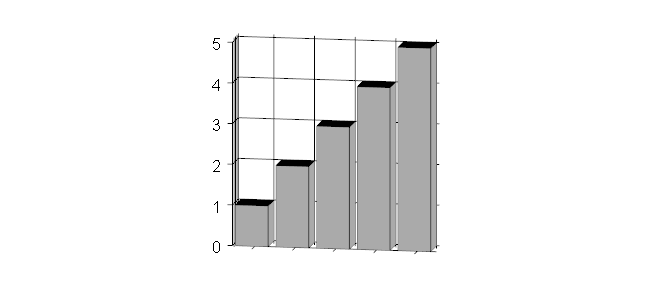### Something more decorative

## Make a prettier 3D barplot with more advanced features
inputdata=round(rnorm(15,10,3))
barplot3d(rows=3,cols=5,z=inputdata,scalexy=5,alpha=0.4,theta=30,phi=50,
topcolors=rainbow(15),xlabels = 1:5,ylabels=LETTERS[1:3],
xsub="Numbers",ysub="Letters",zsub="Count")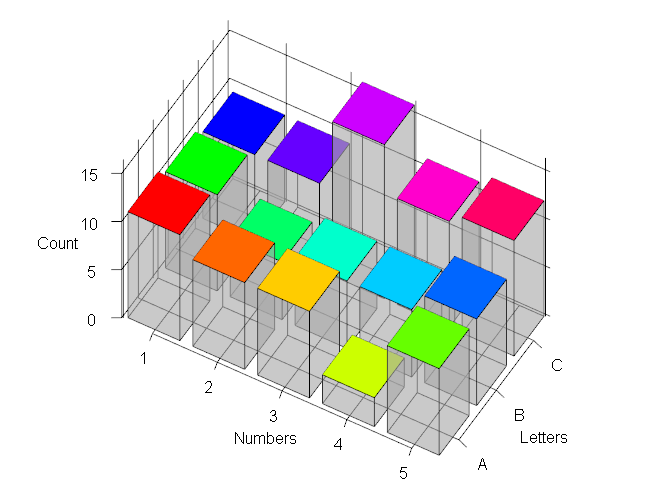## Input data

Input data must be a numeric vector, with one value for each bar you want to plot.

## Setting up your plotting area

The “rows” and “cols” arguments are an essential part of your plot and determine the dimensions of the grid you’re plotting in. However, your data must fit within the plotting area. i.e. 3 rows of 4 bars is 12 bars in total, so don’t attempt to plot 13 bars of data.
NOTE THAT DATA PLOTS LEFT TO RIGHT, FRONT TO BACK. You must consider this in the ordering of your input data.

## Plot 3 rows of 4 bars
barplot3d(rows=3,cols=4,z=1:12,theta=10,phi=10,topcolors=rainbow(12),sidecolors=rainbow(12))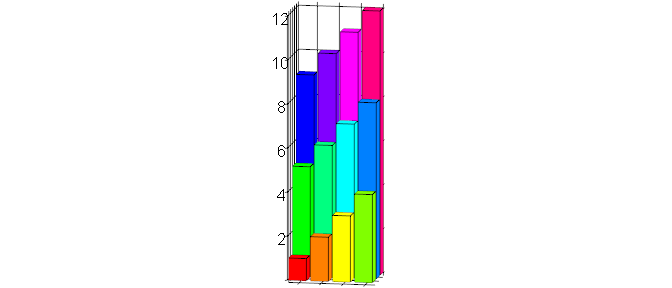## Plot the same data as 2 rows of 6 bars
barplot3d(rows=2,cols=6,z=1:12,theta=10,phi=10,topcolors=rainbow(12),sidecolors=rainbow(12))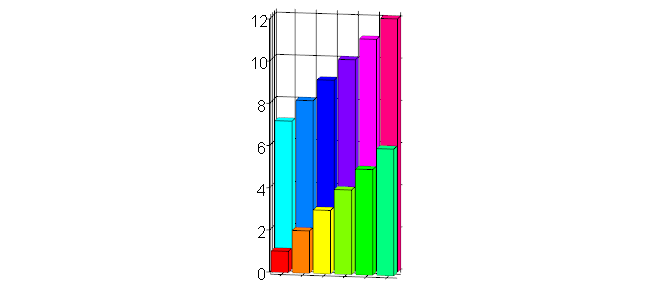## Bar size and gap size between bars

Bars are set to be square (1 unit deep and 1 unit wide), and the gap size is set to be 0.2 units by default. I do not advise changing these values, but if the gap size is adjusted, bear in mind that rendering artifacts can occur if the gap is 0 and the faces of the bars touch one another and are transparent.

## Plot a zero-gapped 3D barplot
barplot3d(rows=1,cols=5,z=1:5,theta=10,phi=10,topcolors = rainbow(5),sidecolors = rainbow(5),gap=0)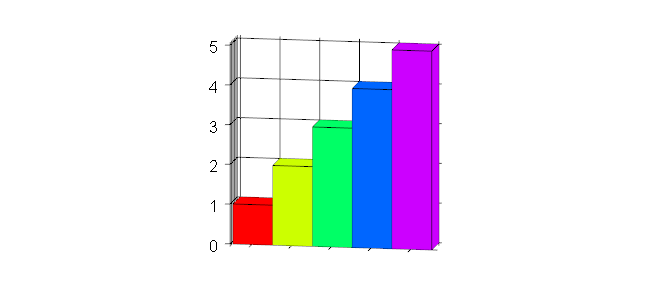The “scalexy” parameter is useful for scaling the size of the bars relative to the Z height. i.e. it can be used to make your plots “skinnier” or “fatter”. Note that this scaling factor does not affect the true values of the data or the values on the Z scale.

## Plot a skinny 3D barplot
barplot3d(rows=2,cols=6,z=1:12,theta=10,phi=10,topcolors=rainbow(12),sidecolors=rainbow(12),scalexy=0.5)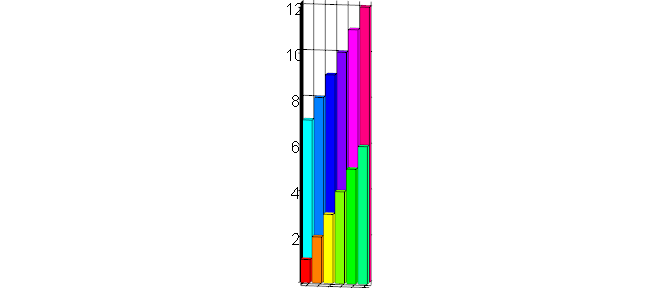## Plot a chubby 3D barplot
barplot3d(rows=2,cols=6,z=1:12,theta=10,phi=10,topcolors=rainbow(12),sidecolors=rainbow(12),scalexy=10)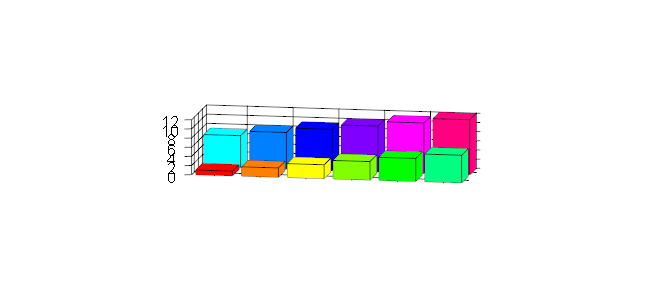## Viewing angles, plot size and saving your plot

All 3D barplots are isometric projections to minimize the potentially misleading effects of perspective. The angle the viewing position is set at is controlled by the “theta” and “phi” parameters. Experiment with these until you find something you like.

• “theta” rotates the viewpoint in the horizontal plane (imagine it on a rotating turntable) and can be set between 0 and 360 degrees.
• “phi” rotates the viewpoint in the vertical plane (imagine going below and above the plot) and can be set between -90 to 90 (-90 is directly below, 90 directly above).

The plot size is determined by the par3d() function; in this vignette, images are captured by a different method. Again, you will have to experiment to find the right settings that make your plot and labels fully visible. I recommend resizing your plot programmatically so that it is completely reproducible. Note that only png format is currently supported by the snapshot function.

# The 4 arguments are the position (in pixels) of the left, top, right and bottom edges of the rgl viewing window
# This code leads to a plot that is 600 pixels wide and 400 pixels tall.
par3d(windowRect=c(0,50,600,450))

# Save the current rgl view
rgl.snapsnot("filename.png")

# If running multiple plots, you should close the viewing window
rgl.close()

## Colors and transparency

Three components of each bar can be colored, and these colors are independent. Each should either be a vector of length 1 (which will be applied to all bars) or a vector the same length as the input data; colors will be recycled if not enough are supplied. Colors can be specified in any way that R natively understands e.g. a simple word such as “red”, an integer or a hexadecimal RGB color, like that returned by rgb() e.g. “#aaaaaa”.

1. “topcolors” specifies the colors of the top of each bar (default is black)
2. “sidecolors” specifies the colors of the sides of each bar (default is gray)
3. “linecolors” specifies the colors of the edges of each bar (default is black)

Transparency of the sides of the bars can be set using the “alpha” parameter. 0 is completely transparent (i.e. a “wireframe” effect) and 1 (the default) is completely opaque. This can be useful when taller bars hide shorter bars, but if used you may want a neutral color like gray so that the topcolors are not altered by the partially transparent colored bar in front of them.

## Plot a partially transparent 3D barplot
barplot3d(rows=2,cols=3,z=1:6,theta=10,phi=10,topcolors=rainbow(6),sidecolors=rev(rainbow(6)),
linecolors=6:1,alpha=0.3)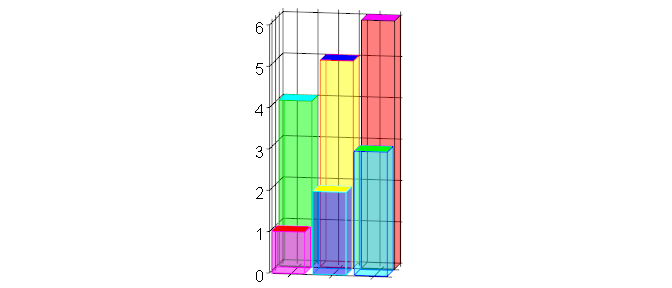## Gridlines and labels

By default there is a grid pattern to make it easier to see the relative height of the bars and add labels to. However, if you want something very plain, you can turn these features on and off as you please, even removing the Z scale entirely. If you don’t like the default way they are implemented, you can explore the source code and use the axis3d() and mtext3d() functions from the rgl package.

## Plot with many labels
barplot3d(rows=2,cols=3,z=1:6,theta=30,phi=50,topcolors=rainbow(6),sidecolors=rainbow(6),
xlabels = c("First","Second","Third"),ylabels=c("Front","Back"),
xsub="Position",ysub="Row",zsub="Z")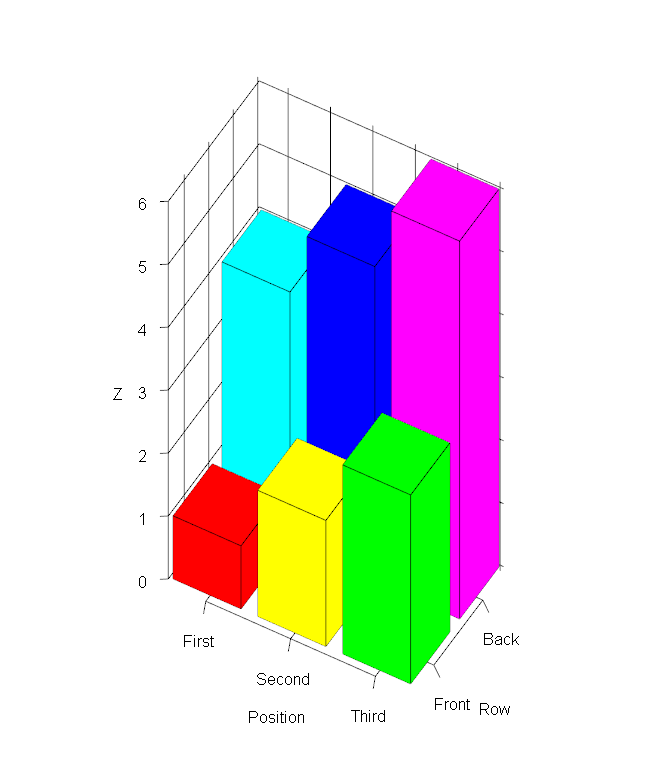## Plot that's extremely plain
barplot3d(rows=2,cols=3,z=1:6,theta=30,phi=50,topcolors=rainbow(6),sidecolors=rainbow(6),
gridlines = FALSE,zlabels=FALSE)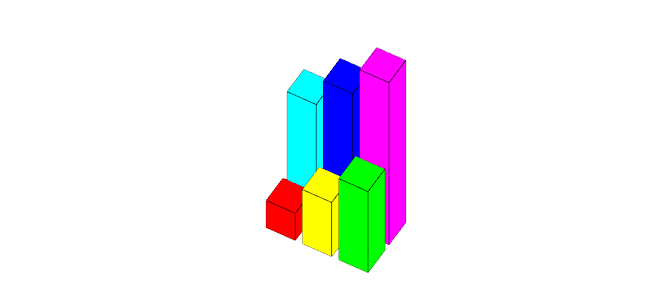# Legoplots

## Introduction and background

These are 3D barplots used to display the counts (or frequency) of the 96 different combinations of somatic mutations and trinucleotide contexts seen in sequencing data. I think their first use was in Figure 1 of Exome and whole genome sequencing of esophageal adenocarcinoma identifies recurrent driver events and mutational complexity by Dulak et al, 2013. These plots are nearly identical, but the preceding and suceeding bases are in the order “ACGT”, not “TCAG”.

## Example usage of legoplot3d()

I have included version 2 of the COSMIC Mutational Signatures. You can use this data to

1. Practice making your own legoplots
2. Checking that your own data is in the same order as the COSMIC data

This cannot be overemphasized: your input data must be in the same order as the COSMIC data i.e. starts with C>A|G>T_AxA and ends with T>G|A>C_TxT. The full list is at the bottom of this vignette, and is stored in the “Somatic_mutation_type” column of the included “signature_probabilities.txt” file.

# Read in COSMIC signature probabilities
x=system.file("extdata", "signature_probabilities.txt", package = "barplot3d")

# Plot signature 2 without axis labels, with Sanger colors and some transparency so we can see all bars
legoplot3d(contextdata=sigdata$Signature_2,labels=FALSE,scalexy=0.05,sixcolors="sanger",alpha=0.4)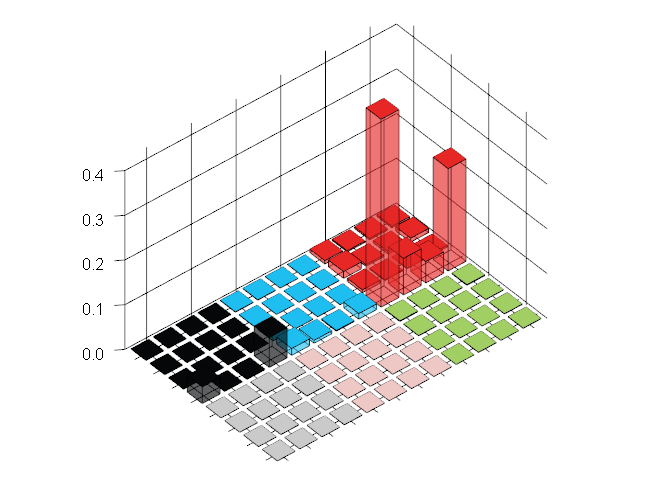Note that you will probably have to play with the “scalexy” parameter to get an appropriately scaled image. Also, the default colors match the Sanger signatures, but you can switch to the original Broad Institute colors if you prefer, or provide 6 arbitrary colors of your own. # Plot signature 2 without axis labels, with Sanger colors and some transparency so we can see all bars legoplot3d(contextdata=sigdata$Signature_8,labels=FALSE,scalexy=0.01,sixcolors="broad",alpha=0.4)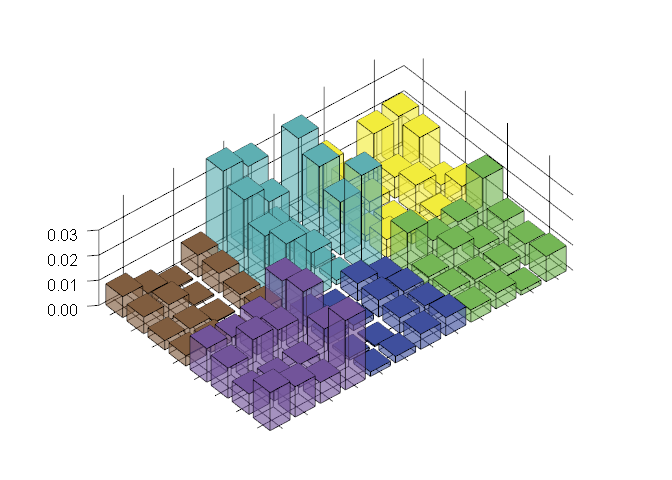# Input data MUST be in this order
cat(sigdata\$Somatic_mutation_type,sep="\n")
#> C>A|G>T_AxA
#> C>A|G>T_CxA
#> C>A|G>T_GxA
#> C>A|G>T_TxA
#> C>A|G>T_AxC
#> C>A|G>T_CxC
#> C>A|G>T_GxC
#> C>A|G>T_TxC
#> C>A|G>T_AxG
#> C>A|G>T_CxG
#> C>A|G>T_GxG
#> C>A|G>T_TxG
#> C>A|G>T_AxT
#> C>A|G>T_CxT
#> C>A|G>T_GxT
#> C>A|G>T_TxT
#> C>G|G>C_AxA
#> C>G|G>C_CxA
#> C>G|G>C_GxA
#> C>G|G>C_TxA
#> C>G|G>C_AxC
#> C>G|G>C_CxC
#> C>G|G>C_GxC
#> C>G|G>C_TxC
#> C>G|G>C_AxG
#> C>G|G>C_CxG
#> C>G|G>C_GxG
#> C>G|G>C_TxG
#> C>G|G>C_AxT
#> C>G|G>C_CxT
#> C>G|G>C_GxT
#> C>G|G>C_TxT
#> C>T|G>A_AxA
#> C>T|G>A_CxA
#> C>T|G>A_GxA
#> C>T|G>A_TxA
#> C>T|G>A_AxC
#> C>T|G>A_CxC
#> C>T|G>A_GxC
#> C>T|G>A_TxC
#> C>T|G>A_AxG
#> C>T|G>A_CxG
#> C>T|G>A_GxG
#> C>T|G>A_TxG
#> C>T|G>A_AxT
#> C>T|G>A_CxT
#> C>T|G>A_GxT
#> C>T|G>A_TxT
#> T>A|A>T_AxA
#> T>A|A>T_CxA
#> T>A|A>T_GxA
#> T>A|A>T_TxA
#> T>A|A>T_AxC
#> T>A|A>T_CxC
#> T>A|A>T_GxC
#> T>A|A>T_TxC
#> T>A|A>T_AxG
#> T>A|A>T_CxG
#> T>A|A>T_GxG
#> T>A|A>T_TxG
#> T>A|A>T_AxT
#> T>A|A>T_CxT
#> T>A|A>T_GxT
#> T>A|A>T_TxT
#> T>C|A>G_AxA
#> T>C|A>G_CxA
#> T>C|A>G_GxA
#> T>C|A>G_TxA
#> T>C|A>G_AxC
#> T>C|A>G_CxC
#> T>C|A>G_GxC
#> T>C|A>G_TxC
#> T>C|A>G_AxG
#> T>C|A>G_CxG
#> T>C|A>G_GxG
#> T>C|A>G_TxG
#> T>C|A>G_AxT
#> T>C|A>G_CxT
#> T>C|A>G_GxT
#> T>C|A>G_TxT
#> T>G|A>C_AxA
#> T>G|A>C_CxA
#> T>G|A>C_GxA
#> T>G|A>C_TxA
#> T>G|A>C_AxC
#> T>G|A>C_CxC
#> T>G|A>C_GxC
#> T>G|A>C_TxC
#> T>G|A>C_AxG
#> T>G|A>C_CxG
#> T>G|A>C_GxG
#> T>G|A>C_TxG
#> T>G|A>C_AxT
#> T>G|A>C_CxT
#> T>G|A>C_GxT
#> T>G|A>C_TxT